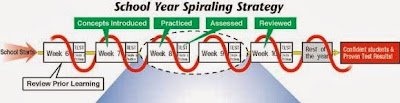## Saturday, November 23, 2013

### Fibonacci Day Math 11-23Happy Fibonacci Day!

Mathematicians celebrate Fibonacci Day on November 23 or 11/23, which are the first four numbers in the Fibonacci sequence shown below.

The Fibonacci Series is a sequence of numbers first created by Leonardo Fibonacci (born in Pisa, Italy) in 1202 and introduced in his writing, Liber abaci.

In this work he introduced the Latin-speaking world to the decimal number system. Later this sequence of numbers was named the Fibonacci sequence in his honor.

The Fibonacci series begins with 0 or 1. After that, use the simple rule: Add the last two numbers to get the next number in the sequence. See the series in action at thinkquest.org.

This is readily translatable into the following set of equations [Liber abaci 3, p4]:
• 1 = 1²
• 1 + 3 = 2²
• 1 + 3 + 5 = 3²
• 1 + 3 + 5 + 7 = 4²
and suggests the general formula:
• 1 + 3 + ... + (2n-1) = n²
From this number statement come the famous Fibonacci numbers:
1, 1, 2, 3, 5, 8, 13, 21, 34, 55, 89, 144, 233, 377, 610, 987, ...

where the first two numbers of the series are 1 and 1 and each number afterward is defined as the sum of the two previous terms, Fn = Fn - 2 + Fn - 1
In Fibonacci's sequence the first number was 1 and the second number was two, the first one was assumed. Read more on our previous blog post, Fibonacci's Spiral and Excel Math

Excel Math also uses a unique spiraling system, similar to that found in the nautilus shell (pictured) to help students retain math concepts over the long term.  \This unique spiraling strategy introduces new math concepts to students while reviewing previously-taught concepts.

Spiraling the math concepts gives students the opportunity to master the old through spaced repetition, while being challenged with the new. Once a concept is introduced, it literally stays in front of the students for the rest of the school year.Excel Math Spiraling Process for math lessons that really work!
This spiraling strategy of repeating concepts at regular intervals throughout the curriculum is an integral part of Excel Math.
"Excel Math allowed me to effectively teach math at two grade levels...without it I don't know how I would have hit all standards and provided the review and practice the students required to succeed.Excel Math provides a solid framework, allowing the teacher to put their own flavor and flare into each lesson or concept. I love Excel Math...it has helped me become a stronger math teacher, and I KNOW it has increased my students' confidence in math (and their test scores!)."
—Anne Evans, Teacher in Idaho

Read more about what teachers, parents and principals around the country are saying about how their students begin to develop a love for math.
Students aren't tested on a subject until they've had multiple chances to succeed in Guided Practice and Homework. Here's a visual road map explaining this spiraling strategy. Excel Math is fully aligned to the Common Core and to state standards. Download correlations here.